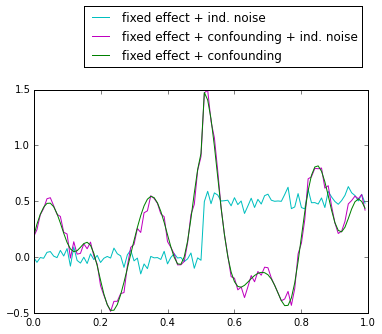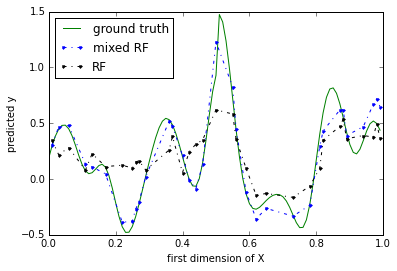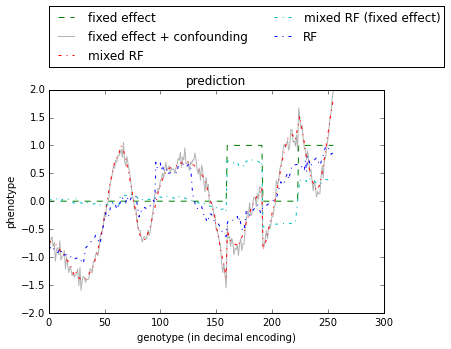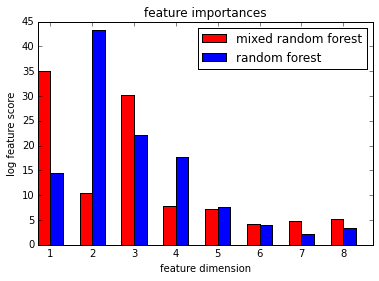# A short guide on how to use mixed random forests¶

## Introduction¶

This document gives a short tutorial on how to use mixed random forest (mixed RF) for feature selection and prediction using python. The reader may benefit from having some background on gaussian process prediction and the general use of the random forest as provided by the python scikit-learn package. However, knowledge of neither is required to follow the steps of this tutorial. All source files for the following examples are to be found in the examples directory of the mixed RF module.

## Examples¶

For each of the following examples we require the loaded mixed RF module, some helper functions, the scipy library and matplotlib for plotting.

In :
# Load limix module for mixed random forest
from limix.ensemble.lmm_forest import  Forest as LMF
from limix.ensemble import lmm_forest_utils as utils
import scipy as sp
import pylab as pl
# Activate inline plotting
%matplotlib inline


### Example 1: Recovering a single fixed effect¶

At first we need to create some data.

In :
sp.random.seed(43)
n_sample = 100
X = sp.empty((n_sample,2))
X[:,0] = sp.arange(0,1,1.0/n_sample)
X[:,1] = sp.random.rand(n_sample)
noise = sp.random.randn(n_sample,1)*.05
y_fixed = (X[:,0:1] > .5)*.5
y_fn = y_fixed + noise


Here we consider a simulated data set of size 100. For each sample we simulate features in 2D where the first dimension is sampled from a grid on [0, 1]. The second dimension contains random samples from the uniform distribution on the interval ]0, 1[. We combine features for all samples into the matrix $\mathbf{X}$. The fixed effect $\mathbf{y}_\text{fixed}$ shall only be affected by the first feature dimension. In addition, we add some gaussian noise to obtain the simulated observation $\mathbf{y}_\text{fn}$.

The second part of the simulation adds structured noise to the observations $\mathbf{y}_\text{fn}$. The samples become connected (i.e. correlated) through the simulated covariance.

In :
# Add some independent noise to make sure kernel is positive semi definite
kernel = utils.getQuadraticKernel(X[:,0], d=0.0025) + sp.eye(n_sample)*1e-8
y_conf = .5*sp.random.multivariate_normal(sp.zeros(n_sample), kernel).reshape(-1,1)
y_tot = y_fn + y_conf


We can now visualize our simulated data (figure 1)

In :
pl.plot(X[:,0], y_fn, 'c')
pl.plot(X[:,0], y_conf+y_fn, 'm')
pl.plot(X[:,0], y_conf + y_fixed, 'g')
pl.legend(['fixed effect + ind. noise',
'fixed effect + confounding + ind. noise',
'fixed effect + confounding'],
bbox_to_anchor=(1.0, 1.4))

Out:
<matplotlib.legend.Legend at 0x7f09387dcb90>Next, we divide our data into training- and test sample.

In :
training_sample = sp.zeros(n_sample, dtype='bool')
training_sample[sp.random.permutation(n_sample)[:sp.int_(.66*n_sample)]] = True
test_sample = ~training_sample
X_train = X[training_sample]
y_train = y_fn[training_sample]


We proceed by fitting the standard random forest to the training sample. We can do so using the mixed RF module with the identity $\mathbf{I}$ as the covariance matrix (i.e. setting kernel=’iid’).

In :
random_forest = LMF(kernel='iid')
random_forest.fit(X[training_sample],y_tot[training_sample])
response_rf = random_forest.predict(X[test_sample])


For fitting the mixed RF we need to pick the rows and colums of the covariance according to the training sample indexes. For prediction we need to use the cross covariance between training and test samples.

In :
kernel_train = kernel[sp.ix_(training_sample, training_sample)]
kernel_test = kernel[sp.ix_(test_sample, training_sample)]
lm_forest = LMF(kernel=kernel_train)
lm_forest.fit(X[training_sample],y_tot[training_sample])
response_lmf = lm_forest.predict(X[test_sample], k=kernel_test)


Finally, we plot the results of our prediction

In :
pl.plot(X[:,0:1], y_fixed + y_conf, 'g')
pl.plot(X[test_sample,0:1], response_lmf, '.b-.')
pl.plot(X[test_sample,0:1], response_rf, '.k-.')
pl.ylabel('predicted y')
pl.xlabel('first dimension of X')
pl.legend(['ground truth', 'mixed RF', 'RF'], loc=2)

Out:
<matplotlib.legend.Legend at 0x7f09386b37d0>### Example 2: Recovering interactions and evaluating feature importances¶

In the second toy example we predict held out simulated data when the phenotype is caused by an interaction of features and the random effect. Now our feature vector is a sorted array of integers ranging from 0 to $2^8−1=255$. We can easily convert this into a 8D feature vector using the binary encoding of each integer, i.e 0 $\rightarrow$ (0, 0, 0, 0, 0, 0, 0, 0) , 1 $\rightarrow$ (0, 0, 0, 0, 0, 0, 0, 1), . . . and 255 $\rightarrow$ (1, 1, 1, 1, 1, 1, 1, 1).

In :
sp.random.seed(42)
n_samples=2**8
x = sp.arange(n_samples).reshape(-1,1)
X = utils.convertToBinaryPredictor(x)


Our simulated fixed effect shall be an interaction of the fist and third feature dimension.

In :
y_fixed = X[:,0:1] * X[:,2:3]


Finally, like in the previous example, we simulate confounding by adding a sample from a multivariate gaussian with a squared quadratic covariance function.

In :
kernel=utils.getQuadraticKernel(x, d=200) + sp.eye(n_samples)*1e-8
y_conf = y_fixed.copy()
y_conf += sp.random.multivariate_normal(sp.zeros(n_samples),kernel).reshape(-1,1)
y_conf += .1*sp.random.randn(n_samples,1)


In addition, we add a small amount of independent gaussian noise. After splitting, we fit, both the mixed RF and the random forest on the training sample. Then test sample is used obtain predictions.

In :
(training, test) = utils.crossValidationScheme(2, n_samples)
lm_forest = LMF(kernel=kernel[sp.ix_(training, training)])
lm_forest.fit(X[training], y_conf[training])
response_tot = lm_forest.predict(X[test], kernel[sp.ix_(test,training)])
# make random forest prediction for comparison
random_forest = LMF(kernel='iid')
random_forest.fit(X[training], y_conf[training])
response_iid = random_forest.predict(X[test])


So far everthing is analog to the previous example. In addition the mixed RF allows us to predict the fixed effect only. All we need to do is dropping the cross covariance from the parameters of the prediction function, i.e

In :
response_fixed = lm_forest.predict(X[test])


The results of all predictions an the ground truth can be observed in the figure below. To visualize the whole binary predictor matrix $\mathbf{X}$ in a single dimension we just need to plot the prediction against the original (decimal) feature vector $\mathbf{x}$.

In :
pl.plot(x, y_fixed, 'g--')
pl.plot(x, y_conf, '.7')
pl.plot(x[test], response_tot, 'r-.')
pl.plot(x[test], response_fixed, 'c-.')
pl.plot(x[test], response_iid, 'b-.')
pl.title('prediction')
pl.xlabel('genotype (in decimal encoding)')
pl.ylabel('phenotype')
pl.legend(['fixed effect', 'fixed effect + confounding',
'mixed RF', 'mixed RF (fixed effect)', 'RF'],
bbox_to_anchor=(1.2, 1.4), ncol=2)

Out:
<matplotlib.legend.Legend at 0x7f0938551f50>Finally, we evaluate the feature importances which are automatically computed while fitting the forests. These scores are plotted in the figure below.

In :
feature_scores_lmf = lm_forest.log_importance
feature_scores_rf = random_forest.log_importance

n_predictors = X.shape
pl.bar(sp.arange(n_predictors), feature_scores_lmf, .3, color='r')
pl.bar(sp.arange(n_predictors)+.3, feature_scores_rf, .3, color='b')
pl.title('feature importances')
pl.xlabel('feature dimension')
pl.ylabel('log feature score')
pl.xticks(sp.arange(n_predictors)+.3, sp.arange(n_predictors)+1)
pl.legend(['mixed random forest', 'random forest'])

Out:
<matplotlib.legend.Legend at 0x7f09384a9910>In [ ]:


In [ ]:


In [ ]: